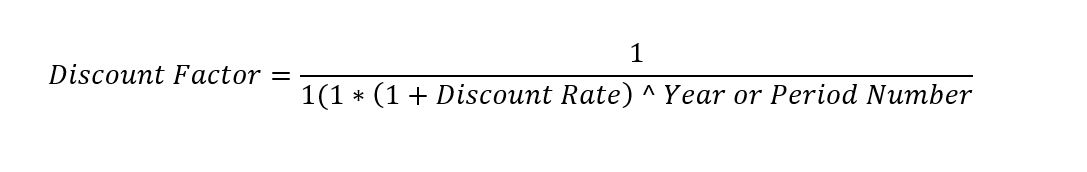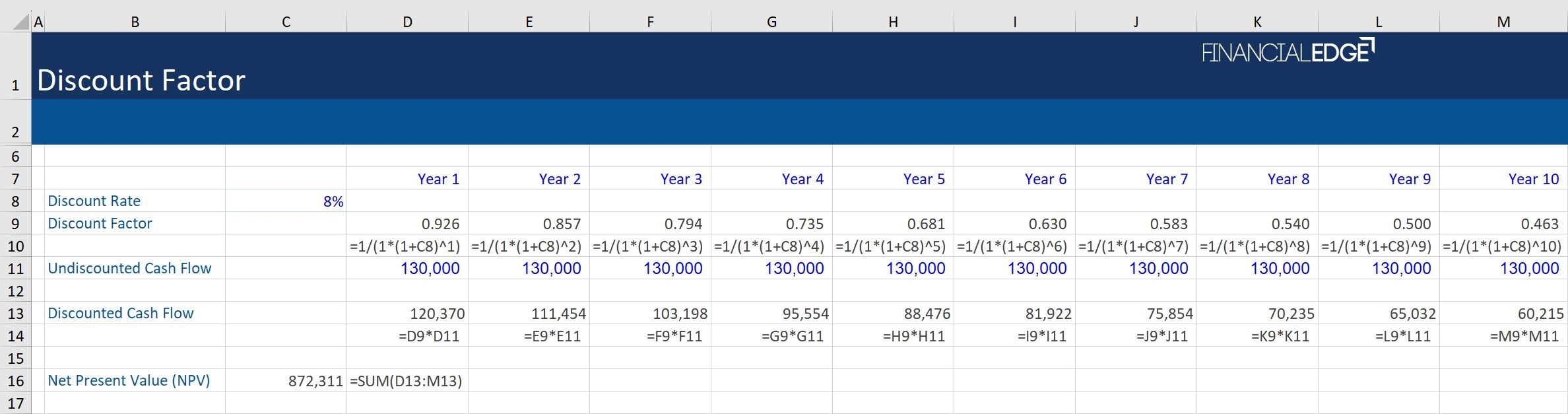## What is a “Discount Factor”?

The term “discount factor” in financial modeling is most commonly used to compute the present value of future cash flows values. It is a weighting factor (or a decimal number) that is multiplied by the future cash flow to discount it to the present value. Simply put, it is a conversion factor when computing the time value of money.

The discount factor is used most commonly when doing valuation using DCF analysis to compute the present value of future cash flow of each period or year. It is also used to calculate the net present value (NPV) which can be used to determine the net future value of an investment. The discount factor is also used by investors in the short-term money market, pension, and insurance companies, and to obtain future investment values.

In financial modeling, once we obtain the undiscounted cash flows for the projected years or the years to come, we need to calculate their present value to evaluate whether the investment is profitable or not and how much is that company worth. The advantage of using the discount factor is that it makes financial modeling more accurate.

## Key Learning Points

• A discount factor is a weighting factor that helps convert future values into the present
• The discount factor is computed through a formula that includes the discount rate (%) and the year or period number (for example year 1 to 10)
• The decimal value of the discount factor gets smaller, as we go along from year 1 to 10 due to the effect of compounding that builds over time

## Discount Factor – Formula

The discount factor is used by analysts when carrying out financial modeling in Excel. The formula to calculate it is stated below:If we are given the discount rate (%) then we can use the aforesaid formula in an Excel spreadsheet to calculate the discount factor for each period (for example, years 1 to 10).

Once we obtain the discount factor for each period, we can multiply the same with the undiscounted cash flow for each period to obtain the discounted cash flow for each period. Thereafter, we derive the total net present value (NPV) of the cash flows – which can be calculated by adding up the individual discounted cash flows for each period (i.e. years 1 to 10). We can also do this by using the NPA formula in excel.

A key point to remember is that to arrive at or obtain the present value of the individual cash flow of any particular period (or year), we multiply the undiscounted cash flow of that period by the discount factor of that period. The choice of the discount rate (%) greatly affects the discount factor value. The discount rate is the rate of return that a project needs to earn to be acceptable to the investor. Using a discount factor enables the analyst to specify the number of days in each period.

Discounting cash flows enables the investor to assess the opportunity cost of undertaking the investment and take into account the time value of money, financing structure, and the risks involved in investing in a particular company vis-à-visor relative to the market.

The discount factor formula is important, as it makes it easier to verify the DCF analysis and obtain more clarity in the net present value (NPV) when conducting financial modeling in excel. The formula enables analysts to view the impact of compounding more clearly for each period.

## Discount Factor Example

Given below is an example, where we first calculate a discount factor for each year (period 1 to 5) and then multiply the same by the undiscounted cash flow value in that year to discount it back to its present value. You will notice that the decimal value of the discount factor gets smaller, as we go along from year 1 to 5. This is due to the effect of compounding that builds over time.(Click to zoom)

Let us assume that each year a company generates US\$130,000 in undiscounted cash flows. We need to calculate the present value for each of these years and then sum them up to get the net present value of the cash flows. For each year we need to use a different discount factor.

In year 5, we use the discount factor formula and obtain the value of the discount factor (0.681). Now, if the undiscounted cash flow in year 5 is \$130,000, then we multiply it by 0.681 to get the present value of this cash flow (discounted cash flow), in this case, \$88,475.8. We do likewise for all years. Thereafter, we calculate the total NPV (\$872, 301.6) by adding all the individual discounted cash flows from year 1 to 10.

Learn to build financial models like an expert with our financial modeling course.